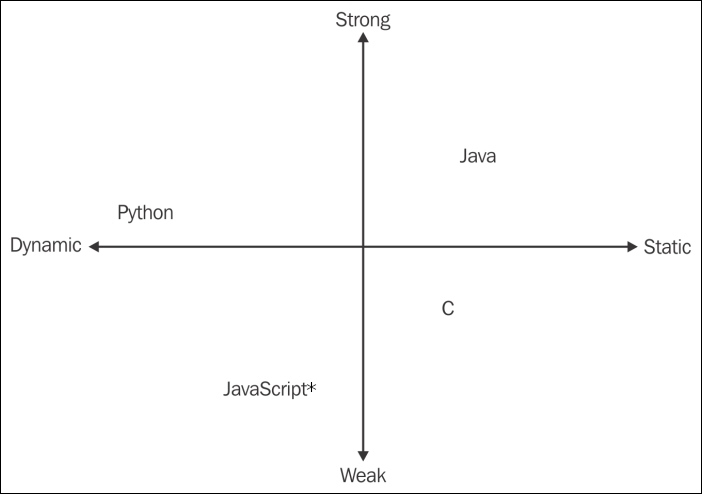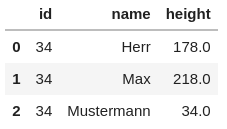Blog

# Pandas DataFrame Validation with Pydantic

With a growing code base and increased intertwinement of classes, methods, and functions, it gets increasingly difficult to deal with the dynamic typing nature of python. Having a clear understanding of what type a function returns (and what input types it requires) helps massively in preventing bugs and being able to adjust the code base quickly and safely without creating bugs. The question is how to keep the great flexibility of the dynamic typing of python while gaining the security and insight from static typing. This article will show a way to validate the output of functions that return Pandas DataFrames.

### Article Structure

We will use the Pydantic package paired with a custom decorator to show a convenient yet sophisticated method of validating functions returning Pandas DataFrames.

The article is structured as follows:

Part 1:

1. Dynamic Typing - We will give a short introduction to dynamic typing and the reasons why output validation is interesting.
2. Pydantic - We will give a short introduction to the Pydantic package.
3. Decorator - We will give a short introduction to decorators.

Part 2:

1. Combining Decorators, Pydantic and Pandas - We will combine points 2. and 3. to showcase how to use them for output validation.
2. Let's define ourselves a proper spaceship! - We will show a complex function DataFrame output with custom and cross column validation taking place.
3. Summary

Readers already familiar with Pydantic classes (e.g. by using FastApi) can skip the first part of section 2, and readers who are already writing custom decorators can skip section 3.

## 1. Dynamic Typing

Python is (luckily) not a statically typed language. That is why we can develop so fast and build the useful code we are writing. The drawback of a dynamically typed language is that we may run into more problems during runtime than we would using a strict static typing scheme. Python will at least throw an error during runtime instead of just casting types all over the place like JavaScript.Question: "Why is JavaScript called a weakly typed language?"
Answer: "Because every week you come into the office and are suprised what type your output will have this week."

Since we prefer to catch a type error before the code runs in production, we are writing tests that check if the result of a function matches the desired type (e.g. via `isinstance(res, str)`). With our extensive test suite we are able to capture a lot of possible bugs and errors before they occur. And we guarantee that a change in the function that breaks any of the expected output will be detected during development.

As I described in the beginning, the reason for these checks is that we are using a dynamically typed language, and we want to keep that speed and flexibility. But we also want the benefits of having a clear understanding what types go into our function, and what types come out of our functions/properties/methods/... . Otherwise, the ever growing codebase will get harder and harder to maintain and to debug.

### Type Annotation

Type annotations are another way to help us see what types should go in and come out of a function, and they allow Pycharm/VScode to give us a highlight if we try to pass a 3 to a function that expects a "3". There are even tools like MyPy which can be incorporated into the CI/CD Jenkins Pipeline to enforce the type annotations and fail the pipeline if there are ints passed to a str parameter.

But type annotations are only a visual help to us / the IDE, they do not actually validate the input/output of functions (and sadly dont speed up the code like in Julia). They are also almost impossible to use together with DataFrames, and most of our functions deal with DataFrames.

### Goals

• flexible code
• robust/stable code in production
• tests/data validation

There are a lot of possibilities to test DataFrames, or the functions returning them. We assert that all columns are there, that all columns have the correct type, that the value ranges are sensible, that the missing values are sensible and so on. And we definitely want these properties to be in the production pipeline too.

By writing unit tests, we can assert all of that, and to make sure the test suite runs in under 3 Minutes, we can use sensible dummy data in all our tests. But we are not asserting all these properties during the production pipeline on the real data.

This is where Pydantic comes in.

## 2. Pydantic

Pydantic is a great tool for input validation, used for example in the FastApi package. Pydantic allows us to define complex data structures and add custom @validator methods that will raise a sensible error message if they are violated.

For example, the following code will validate that any given input to that class fulfills the conditions

• id >= 1
• len(name) <= 20,
• height == None or 0 <= height <= 250.
``````from typing import Optional

from pydantic import BaseModel, Field

class DictValidator(BaseModel):
id: int = Field(..., ge=1)
name: str = Field(..., max_length=20)
height: float = Field(..., ge=0, le=250, description="Height in cm.")``````
``DictValidator(id=1, name='Sebastian', height=178.0)``
``DictValidator(**{"id": 1, "name": "Sebastian", "height": 178.0})``

The next code chunk shows how a sensible error message is returned for an invalid input (here id < 1).

``````from pydantic import ValidationError
# try except so the code chunks run but shows the error raised.
try:
# gives an error because id < 1:
DictValidator(**{"id": 0, "name": "Sebastian", "height": 178.0})
except ValidationError as e:
print(e)``````
``````1 validation error for DictValidator
id
ensure this value is greater than or equal to 1 (type=value_error.number.not_ge; limit_value=1)``````

We see that we can easily validate any dictionary that is given to the Pydantic class.

### Combining Pydantic and Pandas

How does that help us validate DataFrames? By transforming the DataFrames to dict!

`pd.DataFrame().to_dict(orient="records")` will create a dictionary we can pass to the Pydantic class.

``````import pandas as pd
person_data = pd.DataFrame([{"id": 1, "name": "Sebastian", "height": 178},
{"id": 2, "name": "Max", "height": 218},
{"id": 3, "name": "Mustermann", "height": 151}])
#    id        name  height
# 0   1   Sebastian     178
# 1   2         Max     218
# 2   3  Mustermann     151

person_data.to_dict(orient="records")``````
``````[{'id': 1, 'name': 'Sebastian', 'height': 178},
{'id': 2, 'name': 'Max', 'height': 218},
{'id': 3, 'name': 'Mustermann', 'height': 151}]``````

Now we just have to pass each of these dicts to the validator.

We can use a small helper Pydantic class for that:

``````from typing import List
class PdVal(BaseModel):
df_dict: List[DictValidator]

PdVal(df_dict=person_data.to_dict(orient="records"))``````
``PdVal(df_dict=[DictValidator(id=1, name='Sebastian', height=178.0), DictValidator(id=2, name='Max', height=218.0), DictValidator(id=3, name='Mustermann', height=151.0)])``

Now we can build arbitrary complex Pydantic classes that define the content of our DataFrames, and thanks to the `to_dict` transformation, we can use them for validating our data inside the DataFrame on-the-fly while our pipeline is running.

``````# error because height for id 3 is > 250:
wrong_person_data = pd.DataFrame([{"id": 1, "name": "Sebastian", "height": 178},
{"id": 2, "name": "Max", "height": 218},
{"id": 3, "name": "Mustermann", "height": 251}])
#    id        name  height
# 0   1   Sebastian     178
# 1   2         Max     218
# 2   3  Mustermann     251

try:
PdVal(df_dict=wrong_person_data.to_dict(orient="records"))
except ValidationError as e:
print(e)``````
``````1 validation error for PdVal
df_dict -> 2 -> height
ensure this value is less than or equal to 250 (type=value_error.number.not_le; limit_value=250)``````

Now we can save ourselves all the unit tests for shape and form and value ranges etc.

Actually, we are not really saving ourselves from writing the conditions, we just do it differently in a different place and execute the tests every time our function/method/property returns.

Here is a toy example function:

``````# Let's use the user_id as the height for the Mustermann avatar to trigger the validation.
def return_user_avatars(user_id: int) -> pd.DataFrame:
user_avatars = pd.DataFrame(
[
{"id": user_id, "name": "Herr", "height": 178.0},
{"id": user_id, "name": "Max", "height": 218.0},
{"id": user_id, "name": "Mustermann", "height": user_id},
]
)
_ = PdVal(df_dict=user_avatars.to_dict(orient="records"))
return user_avatars``````
``````# works
return_user_avatars(34)````````````1 validation error for PdVal# does not work because height 342 > 250:
try:
return_user_avatars(342)
except ValidationError as e:
print(e)``````
``````df_dict -> 2 -> height
ensure this value is less than or equal to 250 (type=value_error.number.not_le; limit_value=250)``````

Adding `_ = PdVal(df_dict=user_avatars.to_dict(orient="records"))` into every function we write would be tedious, and the `PdVal` class works only with the `id`, `name` and `height` schema.

We need something reusable but also flexible. Luckily, the christmas blog article introduced us to exactly that: reusable and flexible decorators!

What we want is

• to write our function as usual
• then define our DataFrame output shape with the custom validation
• combine the two with as little effort as possible.

So let's write a decorator for that.

To recall what a decorator does check out the christmas decorator blog article.

## 3. Decorator

A decorator takes a function and adds additional functionality around that function without touching the function itself.

Here is the basic definition.

``````def basic_decorator_definition():
def Inner(func):
def wrapper(*args, **kwargs):
res = func(*args, **kwargs)
# or here
return res
return wrapper
return Inner``````

The decorator is a function.

`Inner` takes the function it is decorating as an input.

`wrapper` adds some functionality inside a wrapper function, and then returns the functions result.

(In the christmas blog article the `decorative_christmas_break_timer` did not have the `Inner` function around the wrapper, because we did not pass any input to the decorator. Once we want to do that, we have to add the `Inner` syntax.)

## Summary

In this part of the article we discussed the downsides of python's dynamic typing capabilities in regard to data quality and code maintainability. We gave an introduction into the Pydantic package and showed how decorators work.

In the second part of the article we will see how we can use these concepts for our DataFrame validation.